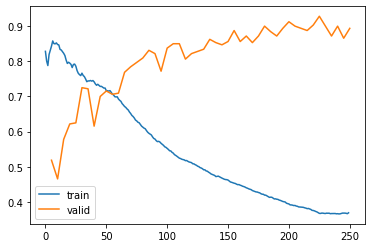In my code, first I change the default callbacks

``````defaults.callbacks = Recorder(train_metrics=True)
defaults.callbacks
``````

to let fastai also plot ‘train metrics’ during training,
here is my Learner:

``````learn = Learner(
dls=dls,
model=model,
loss_func=loss_func,
splitter=my_nn_splitter,
metrics=[
accuracy,
RocAucBinary(),
Recall(),
Precision(),
],
wd_bn_bias=True,
cbs=[
MixUp(),
DataBalanceCallback(),
],
)
learn.to_fp16()
learn.summary()
learn.show_training_loop()
``````

and everything works during fir_one_cycle()

``````learn.fit_one_cycle(
n_epoch=args.epoch,
lr_max=args.lr,
wd=args.wd,
cbs=SaveModelCallback(
monitor='valid_loss',
fname='stage1-bestmodel',
with_opt=False,
),
)
``````

here is the training process

then I run

``````learn.recorder.plot_loss(skip_start=0, with_valid=True)
``````

I got a wrong losses images, because the valid loss is appparently not the values got during my training process. Valid loss should decrease in the second half of process but it didn’tWhy that happened? Is there something wrong in my code?

@SyChen
Your model is overfitting. There’s nothing wrong in your model, except that you’re training for too many epochs. I see you’ve trained this model for 50 epochs. Try a smaller number, say 1 or 2, or 5.

I guess there is something wrong in the loss image, because in the last epoch, valid loss is 0.55, but in the image, the valid loss in the last epoch is ~0.875, so I think this image is incorrect.

I think `learn.recorder.plot_loss` simply plots training loss and the second column of the table, which would normally be `valid_loss`. Here it is plotting `train_loss` and `train_accuracy`.

It looks just like what you said, and I’ve checked the docs, but found nothing useful now.

If you can’t find what you want in the docs you can always jump straight to the code.

``````# https://github.com/fastai/fastai/blob/master/fastai/learner.py
def plot_loss(self, skip_start=5, with_valid=True):
plt.plot(list(range(skip_start, len(self.losses))), self.losses[skip_start:], label='train')
if with_valid:
idx = (np.array(self.iters)<skip_start).sum()
plt.plot(self.iters[idx:], L(self.values[idx:]).itemgot(1), label='valid')
plt.legend()
``````

In your example, changing `itemgot(1)` to `itemgot(5)` and running `plot_loss(learn.recorder)` would give you the plot you want.

Should now be fixed. v2.53
(https://github.com/fastai/fastai/pull/3502)